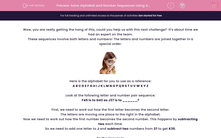# Solve Alphabet and Number Sequences Using Addition and Subtraction

In this worksheet students will solve sequences involving combined letter and number pairs, using addition and subtraction, in both a forward and reverse motion. It will develop students’ problem solving and sequencing skills.This content is premium and exclusive to EdPlace subscribers.Key stage:  KS 2

Curriculum topic:   Verbal Reasoning

Curriculum subtopic:   Letter Connections

Difficulty level:#### Worksheet Overview

Wow, you are really getting the hang of this, could you help us with this next challenge?  It’s about time we had an expert on the team.

These sequences involve both letters and numbers! The letters and numbers are joined together in a special order.Here is the alphabet for you to use as a reference:

A B C D E F G H I J K L M N O P Q R S T U V W X Y Z

Look at the following letter and number pair sequence:

F45 is to G43 as J37 is to ______?

First, we need to work out how the first letter becomes the second letter.

The letters are moving one place to the right in the alphabet.

Now we need to work out how the first number becomes the second number. This happens by subtracting two each time.

So we need to add one letter to J and subtract two numbers from 37 to get K35.

F45 is to G43 as J37 is to K35.Let’s try another:

Z14 is to S11 as K9 is to ______?

First, we need to work out how the first letter becomes the second letter.

The letters are moving seven places to the left in the alphabet.

Now we need to work out how the first number becomes the second number. This happens by subtracting three each time.

So we need to see which letter is seven before K.

and subtract three numbers from 9 to get D6.

Z14 is to S11 as K9 is to D6.

In this activity, you will need to be a sequence superstar to complete the number and letter pairs.